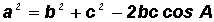POINT OF INTEREST 5 - THE LAW OF COSINESProof of the Law of CosinesThe Law of Cosines is actually a different way of expressing the lengths of the sides of the triangle above.

For example, the length of side a can be found by using the Pythagorean Theorem as followsNotice in the triangle above that we can substitute b cos A for x and b sin A for y.

Therefore,Finally, multiplying out and combining like terms resultsA similar argument can be used for the other two sides and for a triangle having an obtuse angle.